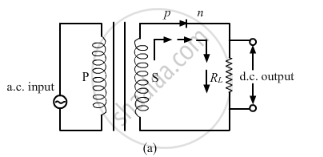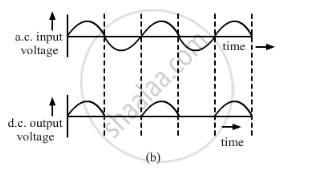# Draw the Circuit Diagram of a Half Wave Rectifier and Explain Its Working. - Physics

Draw the circuit diagram of a half wave rectifier and explain its working.

Explain, with the help of a circuit diagram, the working of a p-n junction diode as a half-wave rectifier.

#### Solution

The circuit diagram for a half wave rectifier is shown below:Working:
During the positive half cycle of the input a.c., the p-n junction is forward biased i.e the forward current flows from p to n and the diode offers a low resistance path to the current. Thus, we get output across-load i.e. a.c input will be obtained as d.c output.

During the negative half cycle of the input a.c., the p-n junction is reversed biased i.e the reverse current flows from n to p, the diode offers a high resistance path to the current. Thus, we get no output across-load. This principle is shown in the diagram given below.Concept: Application of Junction Diode as a Rectifier
Is there an error in this question or solution?